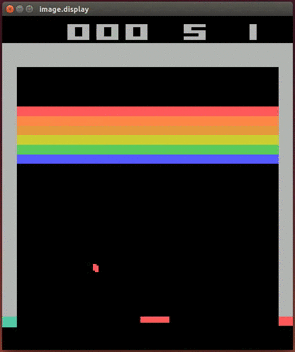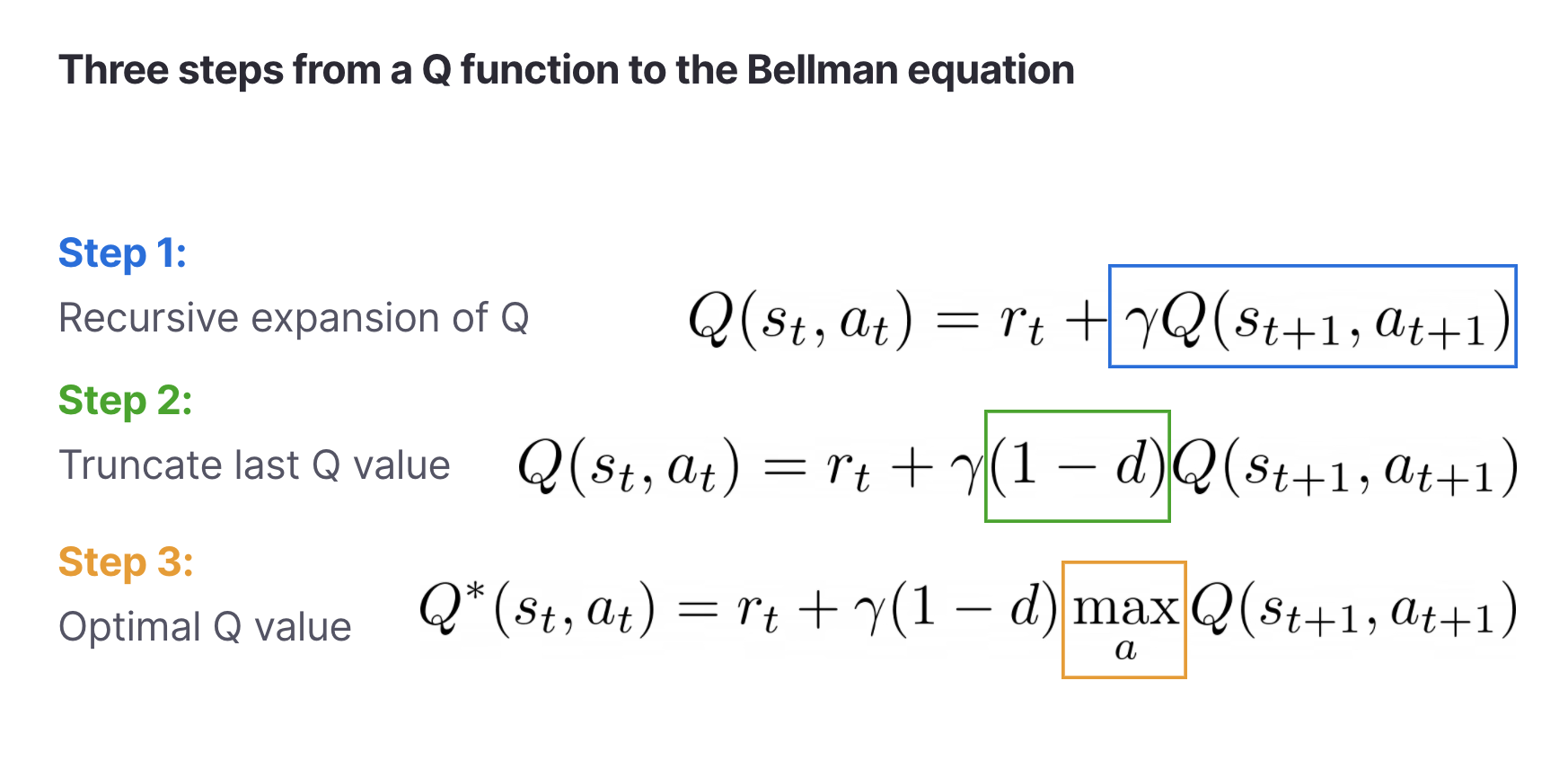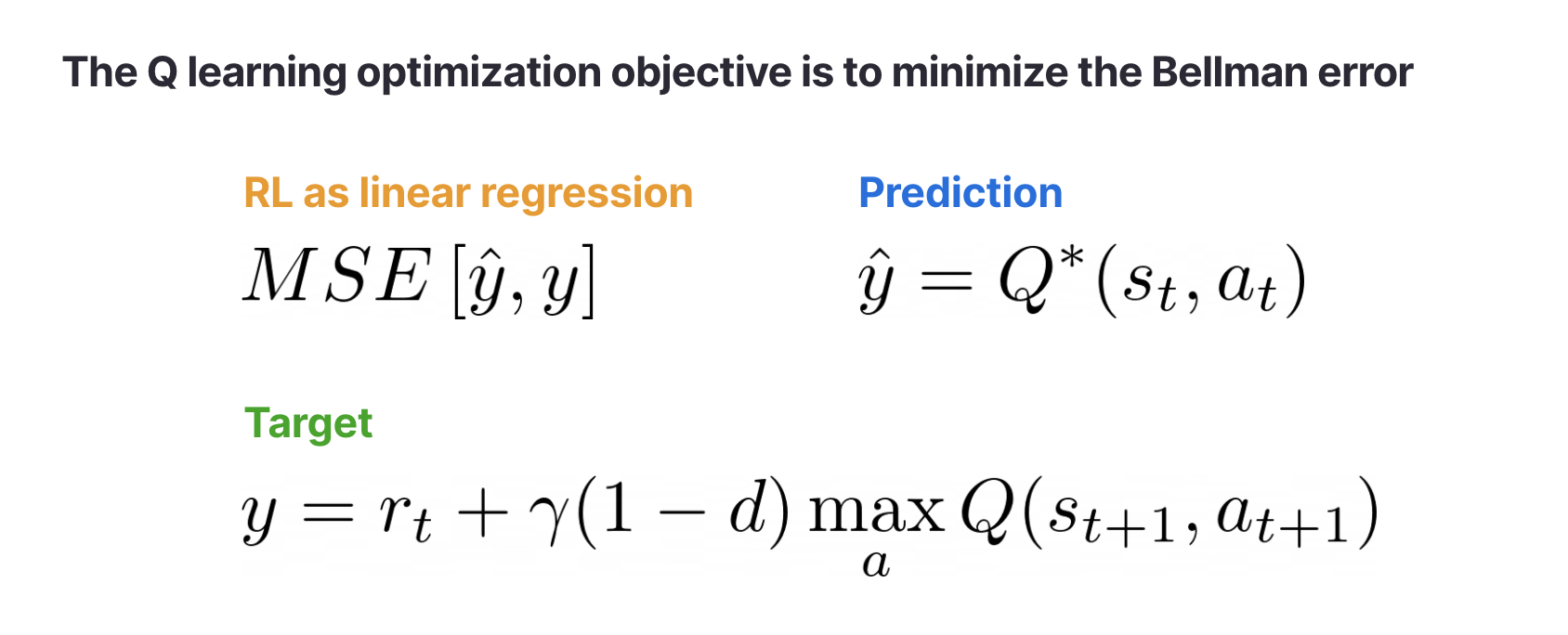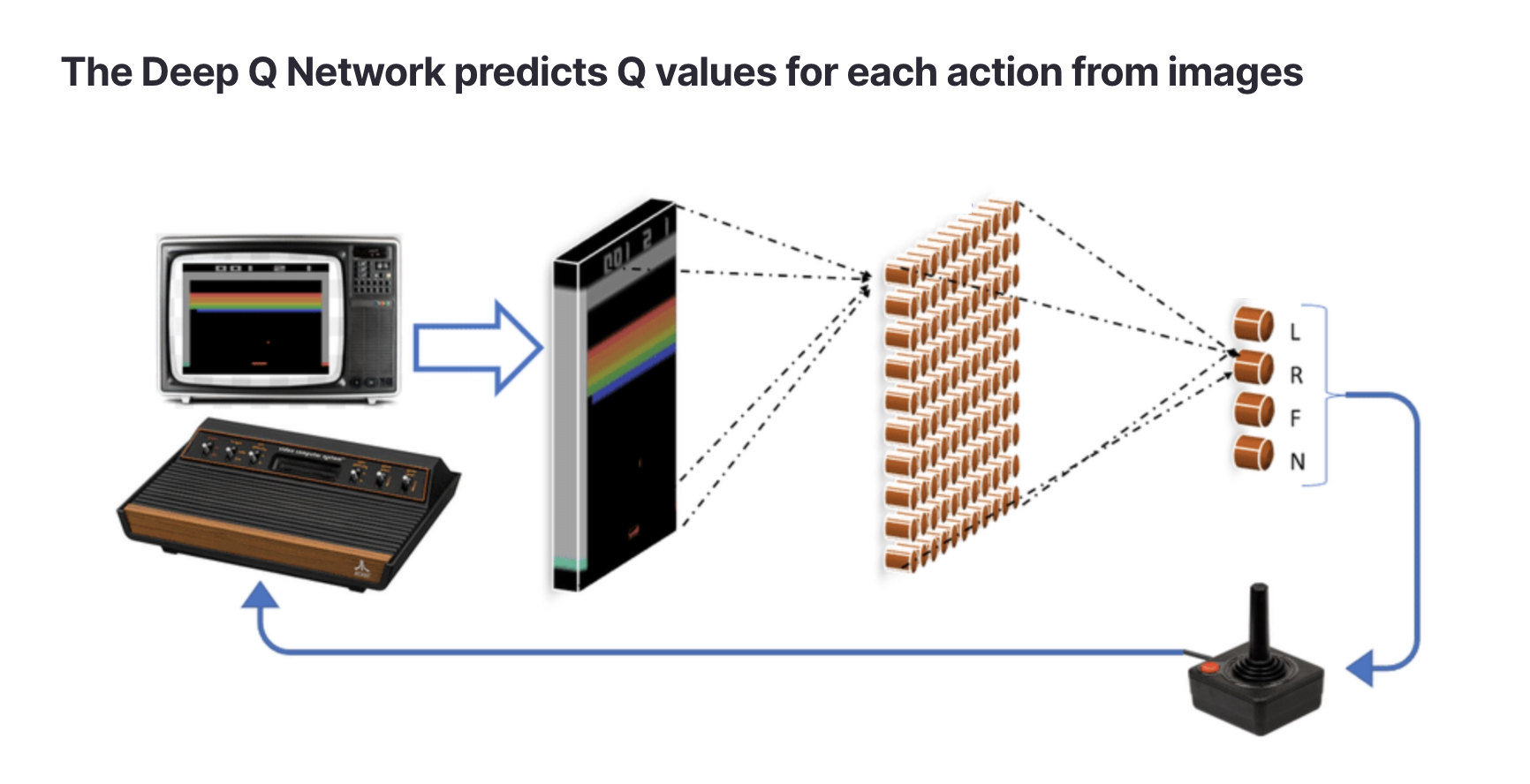# Reinforcement learning with Deep Q Networks

This series on reinforcement learning was guest-authored by Misha Laskin while he was at UC Berkeley. Misha's focus areas are unsupervised learning and reinforcement learning.

In our last posts we introduced reinforcement learning (RL) intuitively, explained why it’s important, and defined a general mathematical framework for building RL agents. As a recap, we defined the goal of RL algorithms in the following way:

💡 The goal of an RL algorithm is to learn a policythat achieves the maximum expected returnsin its environment.

We know that RL algorithms should maximize expected returns, but what objective should they optimize? For a common machine learning problem like regression, we might optimize the model by minimizing the mean squared error (MSE). A neural network that minimizes the MSE will become better at predicting continuous valued targets given some input data. Can we find a similar notion of “error” that when minimized will result in the agent maximizing rewards?

Yes — and to do so we’ll introduce Q functions. A Q function is just another name for the expected returnsand the agent’s goal is to achieve. Q functions are also known as action-value functions because they predict how much return an agent expects to get if it takes a specific action. Intuitively, a Q function says “if I take actionI think I will get a return of.”

In what follows, we’ll derive the Q learning algorithm and show how it was applied to yield one of the first breakthroughs that started the field of Deep RL: the Deep Q Network (DQN). DQNs were the first AI agents to play video games successfully from images. Below is an example of a DQN agent playing the classic game of Breakout.A DQN playing Atari

We still don’t know how a Q function translates to a training objective for RL, but we’re not far from the answer. With a few observations we can derive a loss function for RL. Based on the definitionwhereis a discount factor, we can rewrite a Q function through a following recursive relation. In the below notationis the done flag, which is false for every step except for the last one of the episode, when the episode ends and done is set to true.In step (1) we expanded the sum in the definition of Q. In step (2) we need theterm because at the final step in the episode the Q function is equal to the last reward, since there are no more rewards in the future. In step (3) we noted that the optimal Q valueis achieved by picking the action that will get the highest returns. This is called the Bellman equation.

The leap from this equation to an optimization objective is short. If we want to maximize Q, then we need to make sure that the left and right hand sides of the Bellman equation are equal. So to train an RL algorithm we need to minimize a mean squared error, just like in regression problems!This error is called the Bellman error.

If the state and action spaces are discrete and small, thenis just a table with with states as rows and actions as columns. A tabular Q function can be learned by visiting each state with each action and updating the Q values recursively. But suppose we want to control an autonomous vehicle using image input — then the state space becomes huge (the number of possible images) and we can no longer store Q as a table.

Instead, we’ll approximate Q with a neural network. This is where the “deep” part of Q learning comes in. For simplicity let’s assume that our action space is discrete. Now rather than storing Q as a table, the neural network will input an image (or another type of high dimensional state) and output predictions for the Q values of each action.Source: https://arxiv.org/abs/1903.11012

In torch-like pseudocode a Q network multi-layer perceptron (MLP) might look like this:

``````1
2
3
4
5
6
7
8
9
10
11
"""
A network for predicting Q values
"""
class Qnet:
def __init__(self, action_dim, state_dim, hidden_dim):
# simple MLP that outputs Q values for each action
self.net = nn.Sequential([nn.Linear(state_dim, hidden_dim), nn.ReLU(),
nn.Linear(hidden_dim, hidden_dim), nn.ReLU(),
nn.Linear(hidden_dim, action_dim)])
def forward(self, x):
return self.net(x)``````

For image input, like Atari, we can use a convolutional neural network instead, but in this example we’ll stick with MLPs for simplicity. The Bellman error can be computed as follows:

``````1
2
3
4
5
6
7
8
9
10
11
12
13
14
15
16
"""
Computing the Bellman Error given a transition (s, a, r, s_next, d)
"""
def BellmanError(network, s, a, r, s_next, d, gamma):
# predict Q for all actions from state (s)
all_Q = network(s)
# extract only Q vals for actions taken (a)
Q = all_Q[a]
# compute target Q vals for next state (s_next)
all_target_Q = network(s_next)
# take the highest Q values
target_Q = argmax(all_target_Q, dim=1)
# Bellman eq right hand side
rhs = r + gamma*(1-d)*target_Q
# compute Bellman mean squared error
return mean((Q - rhs)**2)``````

We’ve implemented the Q network and the Bellman error in pseudocode, but where does the transition data `(s, a, r, s_next, d)` come from? During training we run an agent in an environment and the agent stores all transitions it encounters in a database called the replay buffer. For computing the Bellman error we uniformly sample data from the buffer just like we would in normal supervised learning.

``````1
s, a, r, s_next, d = random_transitions_from_buffer(replay_buffer)``````

Finally, we need to specify how our agent chooses its actions when training. The optimal thing to do would be to pick the action with the highest Q value at each state. However, this would result in limited exploration since the agent would always pick the locally optimal action even if exploring would lead it to higher reward regions in the future (i.e., the exploration problem in RL). To ensure that exploration also happens, we can choose to sometimes take random actions and sometimes take optimal ones. This strategy is known as epsilon greedy.

``````1
2
3
4
5
6
7
def epsilon_greedy_step(state, epsilon, action_dim, network):
if uniform(0,1) < epsilon:
# random action
return randint(action_dim)
else:
# greedy action
return argmax(network(state))``````